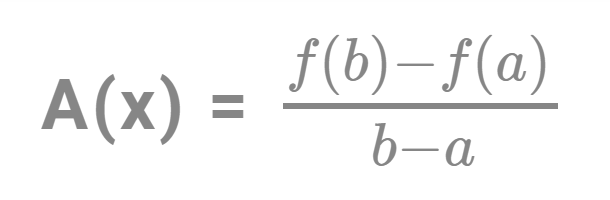# Average Rate of Change Formula

The Average Rate of Change function is defined as the average rate at which one quantity is changing with respect to something else changing. In simple terms, an average rate of change function is a process that calculates the amount of change in one item divided by the corresponding amount of change in another.

### Formula for the Average Rate of Change of a Function

Using function notation, we can define the Average Rate of Change of a function f from a to b as:Where,
f(a) and f(b) are the values of the function f(x) at a and b respectively. Also, a and be are the limits of the function.

### Solved Examples

Question 1: Calculate the average rate of change of a function, f(x) = 3x + 12 as x changes from 5 to 8 .

Solution:

Given,
f(x) = 3x + 12
a = 5
b = 8

f(5) = 3(5) + 12
f(5) = 15 + 12
f(5) = 27

f(8) = 3(8) + 12
f(8) = 24 + 12
f(8) = 36

The average rate of change is,
A(x) =

$$\begin{array}{l}\frac{f(b)-f(a)}{b-a}\end{array}$$

A(x) =

$$\begin{array}{l}\frac{f(8)-f(5)}{8-5}\end{array}$$

A(x) =

$$\begin{array}{l}\frac{36-27}{3}\end{array}$$

A(x) =

$$\begin{array}{l}\frac{9}{3}\end{array}$$

A(x) = 3

Question 2: Calculate the average rate of change of the function f(x) = x2 – 9x in the interval 2 ≤ x ≤ 7.
Solution:
From the given,
f(x) = x2 – 9x
a = 2
b = 7
f(a) = f(2) = (2)2 – 9(2) = 4 – 18 = -14
f(b) = f(7) = (7)2 – 9(7) = 49 – 63 = -14
The average rate of change is:

$$\begin{array}{l}A(x)=\frac{f(b)-f(a)}{b-a}\\=\frac{-14-(-14)}{7-2}\\=\frac{-14+14}{5}\\=0\end{array}$$

Therefore, A(x) = 0